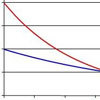#### You may also like### Spinners

How do scores on dice and factors of polynomials relate to each other?### Data Matching

Use your skill and judgement to match the sets of random data.### Into the Exponential Distribution

Get into the exponential distribution through an exploration of its pdf.

# Random Inequalities

### Why do this problem?

This problem requires students to invent a distribution to satisfy certain criteria. In doing so, they will strongly reinforce their understanding of the meaning of distributions as a whole. Students will have to think creatively and really engage with the relationship between a distribution and the probabilities in order to fully solve the problem. The fact that universal order exists underlying all random processes (i.e. the inequalities) is interesting; instead of a collection of diverse results, distributions become part of a coherent whole.

### Possible approach

A main part of this challenge is understanding the meaning of the question. Spend time with the group exploring what they feel that question is asking and encouraging them to work through the meaning of the parts of the question. Once they have a feel for the question they might want to proceed by:
1. Looking at the inequalities for some known distributions (such as the binomial or the normal)
2. Investigating other simple probability distributions (such as that obtained on the roll of a die)
3. Creating distributions 'randomly' using the distribution maker and investigating the inequalities for the probability distributions obtained.

### Key questions

• What is the problem asking?
• How can we use the condition that (??) is a whole number?
• Are then any obvious distributions to try out?

### Possible extension

As a challenge, try to create a distribution which violates the inequalities. How close can you get? Does this give you a feel for why the inequalities hold? How close to the inequalities do distributions which the class already know about (such as binomial or normal) get?

You might like to refer keen students to the central limit theorem in probability, which shows that on average distributions tend to the normal.

### Possible support

Suggest for the first part that students tabulate the probability that X exceeds 1, 2, 3, 4, 5 and 6 for the roll of a single die. Repeat for the roll of 2 dice (these situations are pre-programmed into the distribution maker). Using these explicit results they can explore the inequalities.